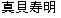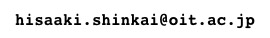\$B??3- \$BA0\$N(Jreview\$B\$X(J / \$B or \$B!V0lHLAjBPO@!!%8%c!<%J%k%/%i%V!WI=;f\$X\$b\$I\$k(J

3\$BBNLdBj\$N?7\$7\$\$0BDj2r(J
reviewed on 2001\$BG/(J6\$B7n(J21\$BF|(J
published...

A New Solution to the Three-Body Problem
Authors: R. Montgomery
Notices of the AMS, May 2001, p471
http://www.ams.org/notices/200105/fea-montgomery.pdf

\$BGX7J(J
(\$BCm0U!'\$3\$l\$O!\$0lHLAjBPO@\$NJ,Ln\$G\$O\$J\$\$!%8EE5NO3X\$G\$"\$k!%(J)
\$B@h=5!\$D+F|?7J9\$K!\$(J3\$BBNLdBj\$N?7\$7\$\$2r\$,H/8+\$5\$l\$?!\$\$H\$\$\$&JsF;\$,\$"\$C\$?\$,!\$(J \$BEv;v \$BO@J8FbMF(J
\$B!!Ey \$B!!\$3\$N2r@b\$K\$h\$k\$H!\$\$b\$H\$b\$H\$3\$N50F;\$NB8:_\$O?tCMu!\$I,\$:\$7\$bBP>N(J \$B\$G\$J\$\$>l9g\$b\$"\$j\$&\$k!K!%\$?\$@\$7!\$(J3\$BBN\$N(J8\$B\$N;z50F;0J30\$O!\$?t3XE*>ZL@\$,\$J\$/!\$(J \$B?tCME*\$K\$b@]F0\$KBP\$7\$FIT0BDj\$@\$=\$&\$@!%(J \$B\$J\$*!\$\$3\$l\$i\$N5DO@\$O\$9\$Y\$F!\$1?F0LL\$r(J2\$Be\$K8BDj\$7\$F\$\$\$k!%(J
\$B!!e5-5-;v!K!\$MW\$9\$k\$K!V(J....\$B!W!%(J

\$BI>(J
\$B!!C1=c\$GLLGr\$/!\$6=L#\$r\$=\$=\$kLdBj\$@\$H;W\$\$\$^\$9!% \$B!!(JN\$BBN\$NF0\$/MM;R\$,!\$(J http://www.maia.ub.es/dsg/\$B\$h\$j!\$%@%&%s%m!<%I\$G\$-\$k\$h\$&\$G\$"\$k(J \$B!J;n\$7\$F\$\$\$J\$\$\$,!K!%(J

added comment on 2002/6/17

\$B>e5-#8\$N;z7?\$N(J3\$BBNLdBj\$N50F;\$K\$D\$\$\$F!\$0BDj@-\$K4X\$9\$kO@J8\$,=P\$?!%(J

Stability and bifurcations of the figure-8 solution of the Three-boday problem
J. Galan, F.J. Munoz-Almaraz, and E. Freire, E. Doedel, and A. Vanderbauwhede
Phys. Rev. Lett. 88, 241101 (2002)
abstract\$B\$O!\$ The stability properties of a recently discovered solution of the general three-body problem with equal masses and the shape of a figure 8 are analyzed as the masses are varied. It is shown by numerical continuation and the evaluation of the characteristic multipliers that the solution is stable only in a narrow mass interval. Other less symmetrical and unstable solutions with equal masses in the same homotopy class as the figure-8 orbit have been found. The branching behavior is also analyzed.

Go back to the top page oforMy current Email address is